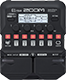# Base Heavy posição 02

Discussion in 'Zoom G1/G1X Four' started by Anderson Scarparo, Apr 10, 2022.

1. Base Heavy posição 02Device: Zoom G1 Four
Firmware: 2.00

Name on device: Base Heavy
Optimized for: Guitar Amp

Effects chain:Base Heavy posição 02

Effect: "TS Drive" (Overdrive / Distortion), active - "yes"
"Gain" = 1
"Boost" = Off
"Tone" = 50
"Volume" = 100

Effect: "RedCrunch" (Overdrive / Distortion), active - "yes"
"Gain" = 50
"Tone" = 72
"Presence" = 48
"Volume" = 70

Effect: "Gt GEQ 7" (Filter), active - "yes"
"100" = 7.5
"200" = 3.5
"400" = 0.0
"800" = 4.0
"1.6k" = 2.5
"3.2k" = 0.0
"6.4k" = -10.0
"VOL" = 75

Effect: "NoiseGate" (Dynamics), active - "yes"
"Detect" = GTRIN
"Depth" = 92
"Threshold" = 41
"Decay" = 0

Effect: "Delay" (Delay), active - "yes"
"Time" = 340
"F.B." = 25
"Mix" = 20
"Tail" = On

Patch Volume: 100